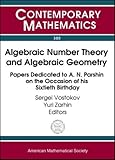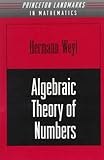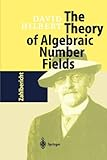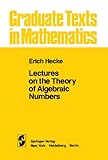Home  - Pure_And_Applied_Math - Algebraic Number Theory
e99.com Bookstore
 Images Newsgroups
 Page 3     41-60 of 134    Back | 1  | 2  | 3  | 4  | 5  | 6  | 7  | Next 20

Algebraic Number Theory:     more books (102)
1. Algebraic K Theory and Algebraic Number Theory: Proceedings of a Seminar Held January 12-16, 1987 With Support from the National Science Foundation (Contemporary Mathematics) by Seminar on Algebraic K-Theory and Algebraic Number Theory, Michael R. Stein, et all 1989-01
2. Algebraic Number Theory (Pure and Applied Mathematics) by R. Long, 1977-09-01
3. Algebraic Number Theory and Algebraic Geometry: Papers Dedicated to A.N. Parshin on the Occasion of His Sixtieth Birthday (Contemporary Mathematics)
4. Algebraic Theory of Numbers by Hermann Weyl, 1998-04-20
5. The Theory of Algebraic Number Fields by David Hilbert, 2010-11-02
6. Lectures on the Theory of Algebraic Numbers (Graduate Texts in Mathematics) by E. T. Hecke, 2010-11-02
7. Algebraic theory of numbers by Pierre Samuel, 1970
8. The Elements Of The Theory Of Algebraic Numbers by Legh Wilber Reid, 2010-09-10
9. Selected Papers on Number Theory and Algebraic Geometry (American Mathematical Society Translations Series 2)
10. Select Topics in Algebra: and Its Interrelations with Logic, Number Theory and Algebraic Geometry (Mathematics and its Applications) by I. Bucur, 1984-09-30
11. Selected Papers on Number Theory, Algebraic Geometry, and Differential Geometry (American Mathematical Society Translations Series 2)
12. Foundations of the theory of algebraic numbers by Harris Hancock, 1964
13. P-Adic Methods in Number Theory and Algebraic Geometry (Contemporary Mathematics) by Steven Sperber, Alan Adolphson, 1992-07
14. The theory of algebraic numbers (Carus mathematical monographs series;no.9) by Harry Pollard, 195041. A Brief Introduction To Classical And Adelic Algebraic Number Theory By William Stein. Text in HTML/DVI/LaTeX/PDF/PS.http://modular.fas.harvard.edu/papers/ant/

43. Algebraic Number Theory
Course notes by Robin Chapman, University of Exeter, May 2000.
http://www.secamlocal.ex.ac.uk/~rjc/courses/ant99/ant99.html
MAS4002: Algebraic Number Theory
This is the home page for the Algebraic Number Theory course. At present it is still under construction. Eventually it will contain copies of course handouts, commentaries on some of my more challenging problems, and useful links. Most files are in dvi format. The course will be closely based on the following book: Ian Stewart and David Tall, Algebraic Number Theory , Chapman and Hall. Alas the paperback is out of print (and the hardback is overpriced) but the following alternative is very cheap (but a bit old-fashioned): Harry Pollard and Harold G. Diamond, The Theory of Algebraic Numbers , Dover. Robin Chapman
Room 811, Laver Building
University of Exeter
Exeter, EX4 4QE, UK
rjc@maths.ex.ac.uk

 44. ALGEBRAIC NUMBER THEORY AND QUADRATIC RECIPROCITY 1. Introduction File Format PDF/Adobe Acrobat Quick Viewhttp://research.microsoft.com/en-us/um/people/cohn/Papers/algnumb.pdf

 45. Algebraic Number Theory - Cambridge University Press Algebraic Number Theory Series Cambridge Studies in Advanced Mathematics (No. 27) A. Fr hlich University of London M. J. Taylor University of Manchester Institute of Sciencehttp://www.cambridge.org/uk/catalogue/catalogue.asp?isbn=0521438349

46. Jan Nekovar's Web Page
Universit Pierre et Marie Curie (Paris VI). Algebraic number theory, arithmetic algebraic geometry.
http://www.math.jussieu.fr/~nekovar/
 Case 247 4, place Jussieu F-75252 PARIS CEDEX 05 FRANCE Tel : Fax: E-mail: nekovar[at]math[.]jussieu[.]fr URL : http://people.math.jussieu.fr/~nekovar/ CV.ps CV.pdf Publications ... Links

 47. ALGEBRAIC NUMBER THEORY 1. Algebraic Integers Let A Be A Subring File Format PDF/Adobe Acrobat Quick Viewhttp://www.fen.bilkent.edu.tr/~franz/ant-st.pdf

48. Proofs Of Quadratic Reciprocity - Wikipedia, The Free Encyclopedia
We are finished because the left hand side is just an alternative expression for (q / p. Proof using algebraic number theory. The proof presented here is by no means the simplest known
edit Proofs that are accessible
Of relatively elementary, combinatorial proofs, there are two which apply types of double counting . One by Ferdinand Eisenstein counts lattice points . Another applies Zolotarev's lemma to Z pqZ expressed by the Chinese remainder theorem as Z pZ Z qZ , and calculates the signature of a permutation
edit Eisenstein's proof
Eisenstein 's proof of quadratic reciprocity is a simplification of Gauss's third proof. It is more geometrically intuitive and requires less technical manipulation. The point of departure is "Eisenstein's lemma", which states that for distinct odd primes p q where denotes the floor function (the largest integer less than or equal to x ), and where the sum is taken over the

49. Nagaraj, D.S.
Institute of Mathematical Sciences, C.I.T., Chennai. Algebraic number theory, algebraic geometry.
http://www.imsc.res.in/~dsn/
I am interested in the following areas of Mathematics: Algebraic Geometry and Algebraic Number theory. IMSc Brochure Hello.
List of publications
• On the moduli of curves with theta-characteristics
Compositio Mathematica, Vol 75, P. 287-297, 1990.
• The stucture of Iwasawa module associated with a \$Z^r_p\$-extension of a p-adic local field of characteristic
Journal of Number theory,Vol 38,No 1, P. 52-57, May 1991.
• (with A.R.Aithal) Splitting types of holomorphic bundles associateted to some hormonic maps.
Comm. In Algebra Vol 21 (10), P. 3727-3731, 1993.
• (with S.Ramanan) Polarisation of type (1,2, ... ,2) on Abelian varieties
Duke Journal of Mathematics, (Pages, 157 - 194), Vol.80, No.1, Oct.1995.
• (with C.S.Seshadri) Degenerations of the moduli spaces of vector bundles on curves I
Proc.Indian Acad. Sci (Math. Sci.), Vol. 107, No. 2, pp 101-137, May 1997.
• (with Indranil Biswas) Parabolic ample bundles, II: Connectivity of zero locus of a class of sections
Topology, Vol. 37, No. 4, pp. 781-789, 1998.
• (with Laytimi Fatima) On the Maximal Degeneracy Loci and the secant vector bundle
Jour. of Math. Sci.(Newyork) 94 (1999), No.1, 1068-1072.

50. Algebraic Number Theory
Schedule Mo 1340 1530, SAZ 02 We 1540 - 1730, SAZ 02 Motivation A standard course in algebraic number theory discusses the proofs of the main results on integral bases
http://www.fen.bilkent.edu.tr/~franz/ant06.html
Schedule
Mo 13:40 - 15:30, SAZ 02 We 15:40 - 17:30, SAZ 02
Motivation
A standard course in algebraic number theory discusses the proofs of the main results on integral bases, discriminants, Dedekind rings, class groups, Dirichlet's unit theorem, etc. In this semester, I will instead concentrate on quadratic extensions of the rationals and of the rational function fields and introduce elliptic curves. This will allow us to do a lot of explicit calculations that cannot be done (by hand) for extensions of higher degree.
Topics
• I. Quadratic Number Fields. Here we will discuss failure of unique factorization, ideal arithmetic, class number computation, and units. Applications: Bachet-Mordell equations y = x +k, some simple 3-descents on elliptic curves like x +y = Az , possibly the cubic reciprocity law.
• II. Quadratic Function Fields. These are quadratic extensions of the rational function fields F p [X]; their arithmetic has a lot in common with quadratic number fields.
• III. Arithmetic of Elliptic and Hyperelliptic Curves. These can essentially be identified with quadratic extensions of function fields.

51. Brown University Mathematics Department
Brown University. Algebraic number theory and topology. Pictures, research papers, notes, transparencies.
http://www.math.brown.edu/faculty/abramovich.html
@import url("http://www.brown.edu/Templates/common/academic/3col-default.css"); @import url("http://www.brown.edu/Templates/common/print/3col-print.css"); @import url("http://www.brown.edu/Templates/common/academic/2-column.css"); @import url("http://www.brown.edu/Templates/common/print/2col-print.css"); @import url("http://www.math.brown.edu/css/math.css"); skip navigation This page is designed for modern browsers. You will have a better experience with a better browser.
• Home People
Box 1917 151 Thayer Street Providence, RI 02912 Telephone: (401) 863-2708 Fax: (401) 863-9013 info@math.brown.edu More Contact Information
Dan Abramovich
Professor of Mathematics
CONTACT INFO
Office: 118 Kassar-Gould House
Phone:
Fax:
Mathematics Department
Box 1917
Brown University
Providence, RI 02912
COURSE SCHEDULE
Spring 2006 Math 252: Algebra
RESEARCH INTERESTS
Algebraic and Arithmetic Geometry
BACKGROUND
Education: Ph.D., Harvard University, 1991.
RECENT PUBLICATIONS
Algebraic orbifold quantum products.

52. GraNTS
GRAduate Number Theory Seminar Algebraic Number Theory and Elliptic Curves Ramblings on how elementary number theory motivates algebraic number theory,
http://www.math.ucdavis.edu/~osserman/seminar/
GRAduate Number Theory Seminar: Algebraic Number Theory and Elliptic Curves
If you are looking for the web page of last Spring's Kolyvagin seminar, you want here instead.
Practical Information
Organizers: Alex Ghitza ( aghitza@math.mit.edu ) and Brian Osserman ( osserman@math.mit.edu
When: Fall 2000, 2 hours/week, MoFr 11-12
Where: MIT, Rm 24-110
Seminar Description
Format: a semester-long seminar giving a rapid introduction to algebraic number theory and elliptic curves. Hopefully, the material will end up including exactly what is needed for an elegant proof of the class number 1 problem for imaginary quadratic extensions, which we will then be able to present at the end of the semester. All participants will be expected to give lectures, and to prepare TeX lecture handouts. Topics: Dedekind domains, rings of integers, scheme-theoretic curves, finite morphisms thereof, splitting and ramification, the Tchebotarov density theorem and class field theory, selected introductory topics from elliptic curve theory, complex multiplication, modular curves, and the solution to Gauss' class number 1 problem. Prerequisites: A semester of graduate algebraic geometry, and familiarity with the commutative algebra required therein.

53. Algebraic Number Theory
Calculus I page. Day Time Monday and Wednesday 210am — 325am. Location Goldsmith 0226
http://people.brandeis.edu/~jbellaic/numbertheory/numbertheory.html
Algebraic Number theory (MATH 203a, Spring 2008)
Location: Goldsmith 0226 Instructor: Joel Bellaiche Contents : Dedekind domains: definition, stability by integral closure, unique factorization of ideals, modules over Dedekind Domain; Extensions of Dedekind domain : discriminant, behaviour of primes; Ring of algebraic numbers of a number field; Finiteness of the class number; Dirichlet units Theorem; Cyclotomic Extensions; Local Fields; Adeles and Ideles, Grossencharacter; Class field theory; Cebotarev Density Theorem; Galois representations Tentative program:
• PART I : Global Theory
• (Algebraic methods) : General theory of Dedekind domains and application to algebraic numbers (2 weeks)
• (Geometric methods) : Bounds on discriminants and finiteness results (Class groups, Dirichlet's Unit Theorem) (2 weeks)
• (Analytic methods) : Zeta and L-functions and applications (Class Number Formula, Dirichlet's prime number theorem, Cebotarev) (2 weeks)
• PART II : Local Theory
• Complete fields, \$p\$-adic fields, completions of number fields (1 week)
• Adeles and Ideles (1 week)
• PART III : Class Field Theory
• Class Field Theory : adelic formulation and consequences (1 week and half)
• Class Field theory : proofs or skecths of the proofs (1 week)
• PART IV : Introduction to the theory of Galois representations
• Grossenchracters and algebraic grossenchracters (1 week)
• Higher dimensional Galois representations (depending on time) Bibliography : We will not follow one specific book. Good references on algebraic number theory abound. Here are some:
• 54. Mathematics Staff Homepage
Manchester. Algebraic number theory, Galois module structure.
http://www.maths.manchester.ac.uk/~mjt/
Sir Martin Taylor FRS
KBE Warden Designate, Merton College Oxford Chair of Royal Society Study, Fruits of Curiosity.
Report: 'The Scientific Century: securing our future prosperity.' Professor in Pure Mathematics Honorary D.Sc. University of Bordeaux 2009 Honorary D.Sc. , University of Leicester 2006 Royal Society Wolfson Research Fellow Qualifications: M.A. (Oxford), Ph.D (London). Room No. 2.106 Administrative duties: Research: Galois, Hermitian and quadratic structure for arithmetic varieties. Publications List CV
Other activities:
Flyfishing, Hill walking. Postal Address: Professor M J Taylor FRS The University of Manchester School of Mathematics Alan Turing Building Oxford Road Manchester, M13 9PL UK Tel: use + 44 161 306 3640 from overseas Fax: Email: Martin.Taylor@manchester.ac.uk

 55. PmWiki - Main - MAA PREP -- Algebraic Number Theory Jul 1, 2010 This is the website for the 2010 MAA PREP course in Algebraic Number Theory. The MAA PREP is funded by the National Science Foundation (NSFhttp://math.bard.edu/cullinan/prep/

56. Math 248B - Algebraic Number Theory - Winter 2004
We conclude by discussing automorphic forms in general and their role in solving fundamental questions in algebraic number theory. It will be sweet.
http://math.stanford.edu/~brubaker/math248b.html
Math 248B - Algebraic Number Theory - Winter 2004
General information Syllabus Homework Handouts
General information
Meeting Time Mon., Wed., Fri., 10:00 - 10:50 (may be negotiated otherwise) Location Building 380 (Math), room 381-T
Professor Ben Brubaker ( brubaker@math.stanford.edu
Office: 382-F (2nd floor, Math building 380)
Office Phone: 3-4507
Office Hours: Monday 2-3:30, Wednesday 2-3:30, or by appointment. Course
Assistant Ryan Vinroot ( vinroot@math.stanford.edu
Office: 380-G (Basement, Math building)
Office hours: By appointment only. Textbooks Multiplicative Number Theory , by Harold Davenport. The Theory of the Riemann Zeta Function , by E.C. Titchmarsh. Course
Content We will review the basics of class field theory in the first week through the lens of reciprocity. Then we'll head to Davenport, covering the first 8 chapters, essential and classical analytic number theory from which all else follows. We'll break and do several weeks on the zeta function. Classical stuff again, but often shoved under the rug. We'll end with a potpourri of topics, including Siegel's theorem and proofs of algebraic facts using analytic information. We conclude by discussing automorphic forms in general and their role in solving fundamental questions in algebraic number theory. It will be sweet. Prerequisites There are essentially no prerequisites for this course. If you took Rubin's 248A in the fall, or know the equivalent information, you'll have a better view from which to understand the arc of the course, but its not at all necessary in understanding the day to day material.

57. Algebraic Number Theory
Algebraic Number Theory From its history as an elegant but abstract area of mathematics algebraic number theory now takes i.
http://www.ramex.com/cr/cr-3696.html
Algebraic Number Theory
Richard Anthony Mollin
CRC Press

Engineering

Algebraic Number Theory

Format
Hardcover
ISBN
Richard Anthony Mollin Algebraic Number Theory email ramex.com Keyword Title Author ISBN ISSN Number Advanced Search Search By Publisher Search By Specialty Search By Media Your Cart is Empty Home About Help Contact ... � 2002 RAmEx Ars Medica, Inc.

Oklahoma State University. Algebraic number theory and algebraic groups, with methods from functional analysis and analytic number theory.
http://www.math.okstate.edu/~wrightd/
Hello:
I am a mathematician on the faculty of Oklahoma State University. My home page is divided up into the following areas of information:
Current Teaching Schedule
My resume and other personal information
Some facts about my education, career and family are given here.
Guide to my published works.
MSRI MathGraphics info: notes and info about lectures
Number Theory and PARI
This a web supplement I created to the textbook Elementary Number Theory and its Applications by Ken Rosen. It contains among other things:
The Indra's Pearls Web Site
Information on a book about kleinian groups which I co-authored and which is now published by Cambridge University Press.
MATH 6490: Indra's Pearls Course Materials
The course ran in the spring of 2004.
Introduction to Dynamical Systems and Fractals
These are the materials I prepared for a course given in the spring of 1996, including a 170-page book processed in latex2html.
Symmetry Web
This is a World Wide Web machine for experimenting with symmetry groups of simple geometric figures.

59. Notes For Dick Gross' Algebraic Number Theory Course
May 25, 1999 As many of you know, I have been typing up the notes for the Number Theory Course being taught by Dick Gross who is visiting from Harvard
http://www.math.utah.edu/~sather/notes.html
As many of you know, I have been typing up the notes for the Number Theory Course being taught by Dick Gross who is visiting from Harvard during the Spring Semester of 1999. I am posting the notes only in .ps and .pdf format since some of the files contain .eps figures. If you need a hard copy or something, email me Before I post anything, I get it approved by Professor Gross, but if you find any mistakes, email me . Cheers. Sean Lectures 1-4 .ps or .pdf : material from 14 January to 26 January Lectures 5-8 .ps or .pdf : material from 28 January to 9 February Lectures 9-12 .ps or .pdf : material from 2 February to 25 February Lectures 13-16 .ps or .pdf : material from 2 March to 11 March Lectures 17-18 .ps or .pdf : material from 23 March to 25 March Lectures 1-18 .ps or .pdf : material from 14 January to 25 March Lectures 1-18 compressed .ps.gz or .pdf.gz : material from 14 January to 25 March Last updated 5.25.99

60. Marcus Wagner
Technische Universit t Berlin. Algebraic number theory.
http://www.math.tu-berlin.de/~wagner/
Marcus Wagner
FB 3 - Mathematik MA 8-1
D-10623 Berlin
Germany phone: 030/314-23761 (MA 812) email: wagner@math.tu-berlin.de
Research Interests:
• Algebraic number theory and function fields
• Algorithmic algebraic number theory and function fields
• Algorithmic applications of algebraic number fields and function fields in cryptography
Thesis, papers and preprints
• On the computation of Hermite-Humbert constants for real quadratic number fields (with M.E. Pohst), Journal de Theorie des Nombres de Bordeaux 17, 2005, 905-920 [ PDF
• , Dissertation, TU-Berlin, 2009 [ PDF
• The algorithm of Cohn revisited (with M.E. Pohst), Preprint 2009 [ PDF
• This table contains 21 Humbert forms which are listed with their minimal vectors, eutactic coefficients and the dimension of perfection. 18 Humbert forms are extreme.
A little course on correspondences
Here is a little course on correspondences which introduces to the theory of correspondences between algebraic function fields. This course is under construction, but you can make first steps with elliptic correspondences in MAGMA.
TU-Berlin